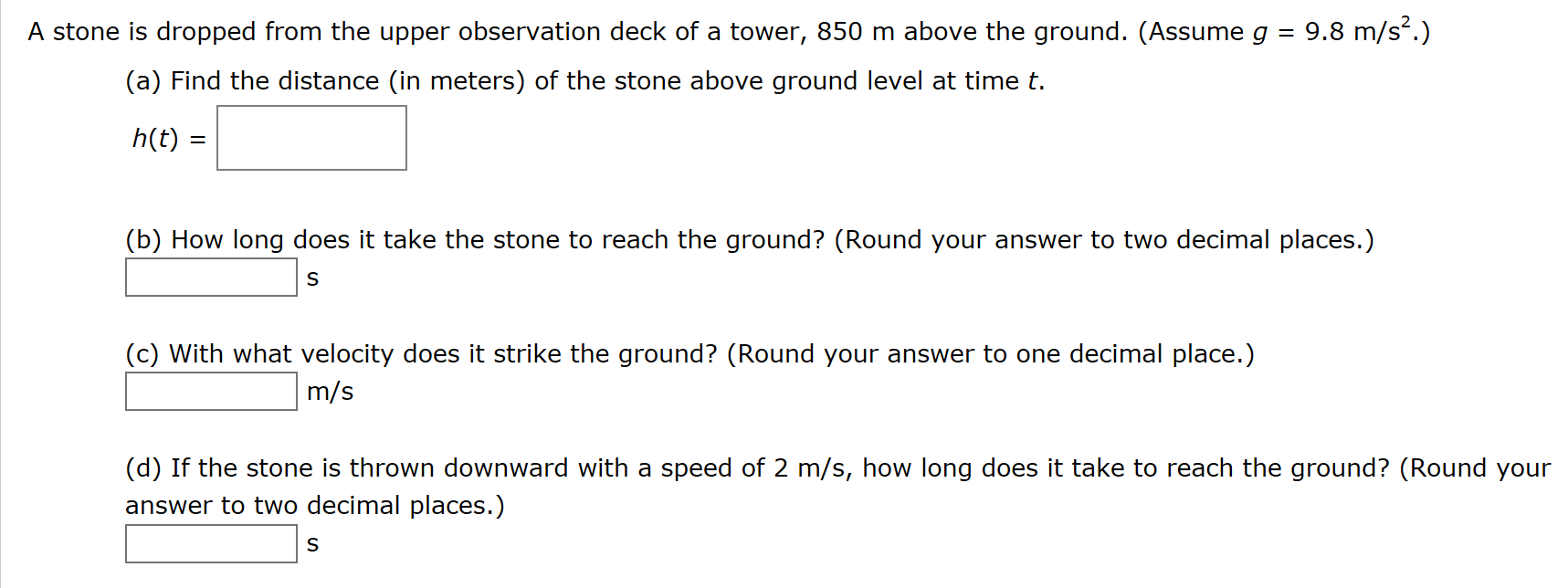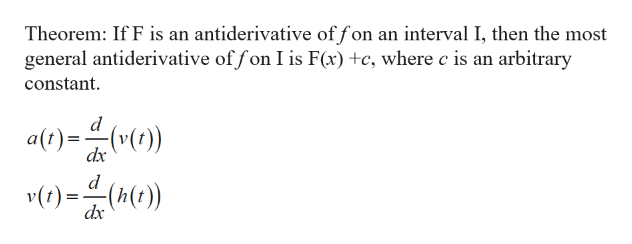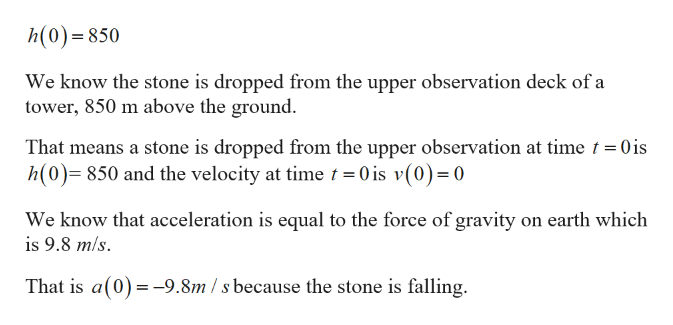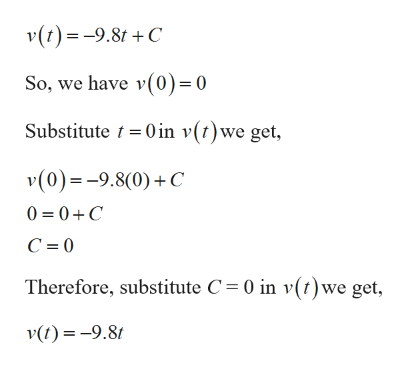# A stone is dropped from the upper observation deck of a tower, 850 m above the ground. (Assume g9.8 m/s2.)(a) Find the distance (in meters) of the stone above ground level at time th(t)(b) How long does it take the stone to reach the ground? (Round your answer to two decimal places.)S(c) With what velocity does it strike the ground? (Round your answer to one decimal place.)m/s(d) If the stone is thrown downward with a speed of 2 m/s, how long does it take to reach the ground? (Round youranswer to two decimal places.)S

Question
140 viewshelp_outlineImage TranscriptioncloseA stone is dropped from the upper observation deck of a tower, 850 m above the ground. (Assume g 9.8 m/s2.) (a) Find the distance (in meters) of the stone above ground level at time t h(t) (b) How long does it take the stone to reach the ground? (Round your answer to two decimal places.) S (c) With what velocity does it strike the ground? (Round your answer to one decimal place.) m/s (d) If the stone is thrown downward with a speed of 2 m/s, how long does it take to reach the ground? (Round your answer to two decimal places.) S fullscreen
check_circle

Step 1

Since you have posted a question with multiple sub-parts, we will solve the first three sub-parts for you. To get remaining sub-part solved please repost the complete question and mention the sub-parts to be solved.

Part (a):

To find the distance of the stone above the ground level at time thelp_outlineImage TranscriptioncloseTheorem: If F is an antiderivative of fon an interval I, then the most general antiderivative of fon I is F(x) +c, where c is an arbitrary constant d alt)()) dx d v() dx fullscreen
Step 2

Given,help_outlineImage Transcriptioncloseh(0)=850 We know the stone is dropped from the upper observation deck of a tower, 850 m above the ground That means a stone is dropped from the upper observation at time t 0 is h(0)=850 and the velocity at time t 0 is v(0) 0 We know that acceleration is equal to the force of gravity on earth which is 9.8 m/s That is a(0)9.8m /s because the stone is falling = fullscreen
Step 3

The general antiderivative of a(t) using the...help_outlineImage Transcriptionclosev(t)9.8tC So, we have v(0) 0 Substitute t 0in v(t)we get, v(0)=-9.8(0)C 0 0C С %3D0 Therefore, substitute C = 0 in v(t)we get v(t)-9.8 fullscreen

### Want to see the full answer?

See Solution

#### Want to see this answer and more?

Solutions are written by subject experts who are available 24/7. Questions are typically answered within 1 hour.*

See Solution
*Response times may vary by subject and question.
Tagged in

### Other